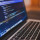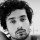Tough Puzzles & Brain Teasers
Puzzles on logic and mathematics that will make you scratch your head.
79 Members
Join this group to post and comment.Aswini Vi
Computer Science
14 Jan 2019

# What will be the value of x in 1,4,14,45,X,422,1272 ?

Find the value of X in the below sequence, 1,4,14,45,X,422,1272 ?Ankita Katdare
Computer Science
4mos ago

I think the answer for this will be 139

The next number is 3 times the previous number + variable n. Here the variable n increases by 1 after each entry.

After 1,

4 = 3 x 1 + 1
14 = 3 x 4 + 2
45 = 3 * 14 + 3
139 = 3 x 45 + 4
422 = 3 x 139 + 5
1272 = 3 * 422 + 6Aswini Vi
Computer Science
4mos ago

Your answer is correct.Thanks for clear explanationRohit Kumar
Electronic
4mos ago

What is the way to solve such questions?? 😕